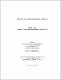## Minimization of lines in reversible circuits2015
##### Authors
Law, Jayati J.
University of Lethbridge. Faculty of Arts and Science
##### Publisher
Lethbridge, Alta. : University of Lethbridge, Dept. of Mathematics and Computer Science
##### Abstract
Reversible computing has been theoretically shown to be an efficient approach over conventional computing due to the property of virtually zero power dissipation. A major concern in reversible circuits is the number of circuit lines or qubits which are a limited resource. In this thesis we explore the line reduction problem using a decision diagram based synthesis approach and introduce a line reduction algorithm— Minimization of lines using Ordered Kronecker Functional Decision Diagrams (MOKFDD). The algorithm uses a new sub-circuit for a positive Davio node structure in addition to the existing node structures. We also present a shared node ordering for OKFDDs. OKFDDs are a combination of OBDDs and OFDDs. The experimental results shows that the number of circuit lines and quantum cost can be reduced with our proposed approach.
##### Keywords
Ordered Kronecker Functional Decision Diagrams , OKFDDs , reversible logic , Boolean function , logic synthesis , line reduction